# 24.3 Basicity of Amines

$$\newcommand{\vecs}{\overset { \rightharpoonup} {\mathbf{#1}} }$$ $$\newcommand{\vecd}{\overset{-\!-\!\rightharpoonup}{\vphantom{a}\smash {#1}}}$$$$\newcommand{\id}{\mathrm{id}}$$ $$\newcommand{\Span}{\mathrm{span}}$$ $$\newcommand{\kernel}{\mathrm{null}\,}$$ $$\newcommand{\range}{\mathrm{range}\,}$$ $$\newcommand{\RealPart}{\mathrm{Re}}$$ $$\newcommand{\ImaginaryPart}{\mathrm{Im}}$$ $$\newcommand{\Argument}{\mathrm{Arg}}$$ $$\newcommand{\norm}{\| #1 \|}$$ $$\newcommand{\inner}{\langle #1, #2 \rangle}$$ $$\newcommand{\Span}{\mathrm{span}}$$ $$\newcommand{\id}{\mathrm{id}}$$ $$\newcommand{\Span}{\mathrm{span}}$$ $$\newcommand{\kernel}{\mathrm{null}\,}$$ $$\newcommand{\range}{\mathrm{range}\,}$$ $$\newcommand{\RealPart}{\mathrm{Re}}$$ $$\newcommand{\ImaginaryPart}{\mathrm{Im}}$$ $$\newcommand{\Argument}{\mathrm{Arg}}$$ $$\newcommand{\norm}{\| #1 \|}$$ $$\newcommand{\inner}{\langle #1, #2 \rangle}$$ $$\newcommand{\Span}{\mathrm{span}}$$$$\newcommand{\AA}{\unicode[.8,0]{x212B}}$$

Objectives

After completing this section, you should be able to

1. account for the basicity and nucleophilicity of amines.
2. explain why amines are more basic than amides, and better nucleophiles.
3. describe how an amine can be extracted from a mixture that also contains neutral compounds illustrating the reactions which take place with appropriate equations.
4. explain why primary and secondary (but not tertiary) amines may be regarded as very weak acids, and illustrate the synthetic usefulness of the strong bases that can be formed from these weak acids.
##### Key Terms

Make certain that you can define, and use in context, the key term below.

• amide
##### Study Notes

The lone pair of electrons on the nitrogen atom of amines makes these compounds not only basic, but also good nucleophiles. Indeed, we have seen in past chapters that amines react with electrophiles in several polar reactions (see for example the nucleophilic addition of amines in the formation of imines and enamines in Section 19.8).

The ammonium ions of most simple aliphatic amines have a pKa of about 10 or 11. However, these simple amines are all more basic (i.e., have a higher pKa) than ammonia. Why? Remember that, relative to hydrogen, alkyl groups are electron releasing, and that the presence of an electron‑releasing group stabilizes ions carrying a positive charge. Thus, the free energy difference between an alkylamine and an alkylammonium ion is less than the free energy difference between ammonia and an ammonium ion; consequently, an alkylamine is more easily protonated than ammonia, and therefore the former has a higher pKa than the latter.

## Basicity of nitrogen groups

In this section we consider the relative basicity of amines. When evaluating the basicity of a nitrogen-containing organic functional group, the central question we need to ask ourselves is: how reactive (and thus how basic and nucleophilic) is the lone pair on the nitrogen? In other words, how much does that lone pair want to break away from the nitrogen nucleus and form a new bond with a hydrogen. The lone pair electrons makes the nitrogen in amines electron dense, which is represents by a red color in the electrostatic potential map present below left. Amine are basic and easily react with the hydrogen of acids which are electron poor as seen below.Amines are one of the only neutral functional groups which are considered bases which is a consequence of the presence of the lone pair electrons on the nitrogen. During an acid/base reaction the lone pair electrons attack an acidic hydrogen to form a N-H bond. This gives the nitrogen in the resulting ammonium salt four single bonds and a positive charge.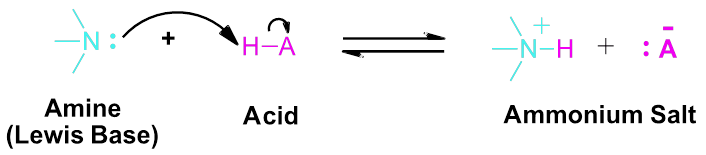Amines react with water to establish an equilibrium where a proton is transferred to the amine to produce an ammonium salt and the hydroxide ion, as shown in the following general equation:

$RNH2_{(aq)}+H_2O_{(l)} \rightleftharpoons RNH3^+_{(aq)}+OH^−_{(aq)} \label{16.5.4}$

The equilibrium constant for this reaction is the base ionization constant (Kb), also called the base dissociation constant:

$K_b=\dfrac{[RNH3^+][OH^−]}{[NH2]} \label{16.5.5}$

pKb = -log Kb

Just as the acid strength of a carboxylic acid can be measured by defining an acidity constant Ka (Section 2-8), the base strength of an amine can be mea­sured by defining an analogous basicity constant Kb. The larger the value of Kb and the smaller the value of pKb, the more favorable the proton-transfer equi­librium and the stronger the base.

However, Kb values are often not used to discuss relative basicity of amines. It is common to compare basicity's of amines by using the Ka's of their conjugate acids, which is the corresponding ammonium ion. Fortunately, the Ka and Kb values for amines are directly related.

Consider the reactions for a conjugate acid-base pair, RNH3+ − RNH2:

$\ce{RNH3+}(aq)+\ce{H2O}(l)⇌\ce{RNH2}(aq)+\ce{H3O+}(aq) \hspace{20px} K_\ce{a}=\ce{\dfrac{[RNH2][H3O]}{[RNH3+]}}$

$\ce{RNH2}(aq)+\ce{H2O}(l)⇌\ce{RNH3+}(aq)+\ce{OH-}(aq) \hspace{20px} K_\ce{b}=\ce{\dfrac{[RNH3+][OH-]}{[RNH2]}}$

Adding these two chemical equations together yields the equation for the autoionization for water:

$\cancel{\ce{RNH3+}(aq)}+\ce{H2O}(l)+\cancel{\ce{RNH2}(aq)}+\ce{H2O}(l)⇌\ce{H3O+}(aq)+\cancel{\ce{RNH2}(aq)}+\ce{OH-}(aq)+\cancel{\ce{RNH3+}(aq)}$

$\ce{2H2O}(l)⇌\ce{H3O+}(aq)+\ce{OH-}(aq)$

Given that the K expression for a chemical equation formed from adding two or more other equations is the mathematical product of the input equations’ K constants.

Ka X Kb = {2 H2O} / (H3O+}{OH-} = Kw

$K_\ce{a}=\dfrac{K_\ce{w}}{K_\ce{b}}$

pKa + pKb =14

Thus if the Ka for an ammonium ion is know the Kb for the corresponding amine can be calculated using the equation Kb = Kw / Ka. This relationship shows that as an ammonium ion becomes more acidic (Ka increases / pKa decreases) the correspond base becomes weaker (Kb decreases / pKb increases)

Weaker Base = Larger Ka and Smaller pKa of the Ammonium ion

Stronger Base = Smaller Ka and Larger pKa of the Ammonium ion

Like ammonia, most amines are Brønsted-Lowry and Lewis bases, but their base strength can be changed enormously by substituents. Most simple alkyl amines have pKa's in the range 9.5 to 11.0, and their aqueous solutions are basic (have a pH of 11 to 12, depending on concentration).

Aromatic herterocyclic amines (such as pyrimidine, pyridine, imidazole, pyrrole) are significantly weaker bases as a consequence of three factors. The first of these is the hybridization of the nitrogen. In each case the heterocyclic nitrogen is sp2 hybridized. The increasing s-character brings it closer to the nitrogen nucleus, reducing its tendency to bond to a proton compared to sp3 hybridized nitrogens. The very low basicity of pyrrole reflects the exceptional delocalization of the nitrogen electron pair associated with its incorporation in an aromatic ring. Imidazole (pKa = 6.95) is over a million times more basic than pyrrole because the sp2 nitrogen that is part of one double bond is structurally similar to pyridine, and has a comparable basicity.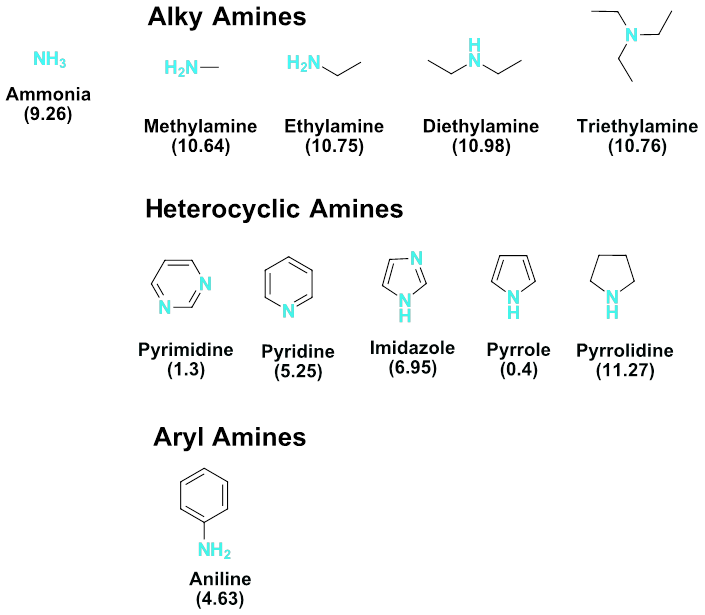Basicity of common amines (pKa of the conjugate ammonium ions)

## Inductive Effects in Nitrogen Basicity

Alkyl groups donate electrons to the more electronegative nitrogen. The inductive effect makes the electron density on the alkylamine's nitrogen greater than the nitrogen of ammonia. The small amount of extra negative charge built up on the nitrogen atom makes the lone pair even more attractive towards hydrogen ions. Correspondingly, primary, secondary, and tertiary alkyl amines are more basic than ammonia.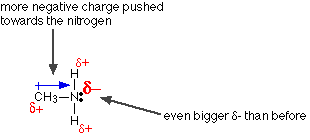Compound pKa of the conjugate acid NH3 9.3 CH3NH2 10.66 (CH3)2NH 10.74 (CH3)3N 9.81

## Comparing the Basicity of Alkylamines to Amides

The nitrogen atom is strongly basic when it is in an amine, but not significantly basic when it is part of an amide group. While the electron lone pair of an amine nitrogen is localized in one place, the lone pair on an amide nitrogen is delocalized by resonance. The electron density – in the form of a lone pair – is stabilized by resonance delocalization, even though there is not a negative charge involved. Here’s another way to think about it: the lone pair on an amide nitrogen is not as available for bonding with a proton – these two electrons are too stable being part of the delocalized pi-bonding system. The electrostatic potential map shows the effect of resonance on the basicity of an amide. The map shows that the electron density, shown in red, is almost completely shifted towards the oxygen. This greatly decreases the basicity of the lone pair electrons on the nitrogen in an amide.Comparison of amines and amides to rationalize the the pKa values of their conjugate acids

## Amine Extraction in the Laboratory

Extraction is often employed in organic chemistry to purify compounds. Liquid-liquid extractions take advantage of the difference in solubility of a substance in two immiscible liquids (e.g. ether and water). The two immiscible liquids used in an extraction process are (1) the solvent in which the solids are dissolved, and (2) the extracting solvent. The two immiscible liquids are then easily separated using a separatory funnel. For amines one can take advantage of their basicity by forming the protonated salt (RNH2+Cl), which is soluble in water. The salt will extract into the aqueous phase leaving behind neutral compounds in the non-aqueous phase. The aqueous layer is then treated with a base (NaOH) to regenerate the amine and NaCl. A second extraction-separation is then done to isolate the amine in the non-aqueous layer and leave behind NaCl in the aqueous layer.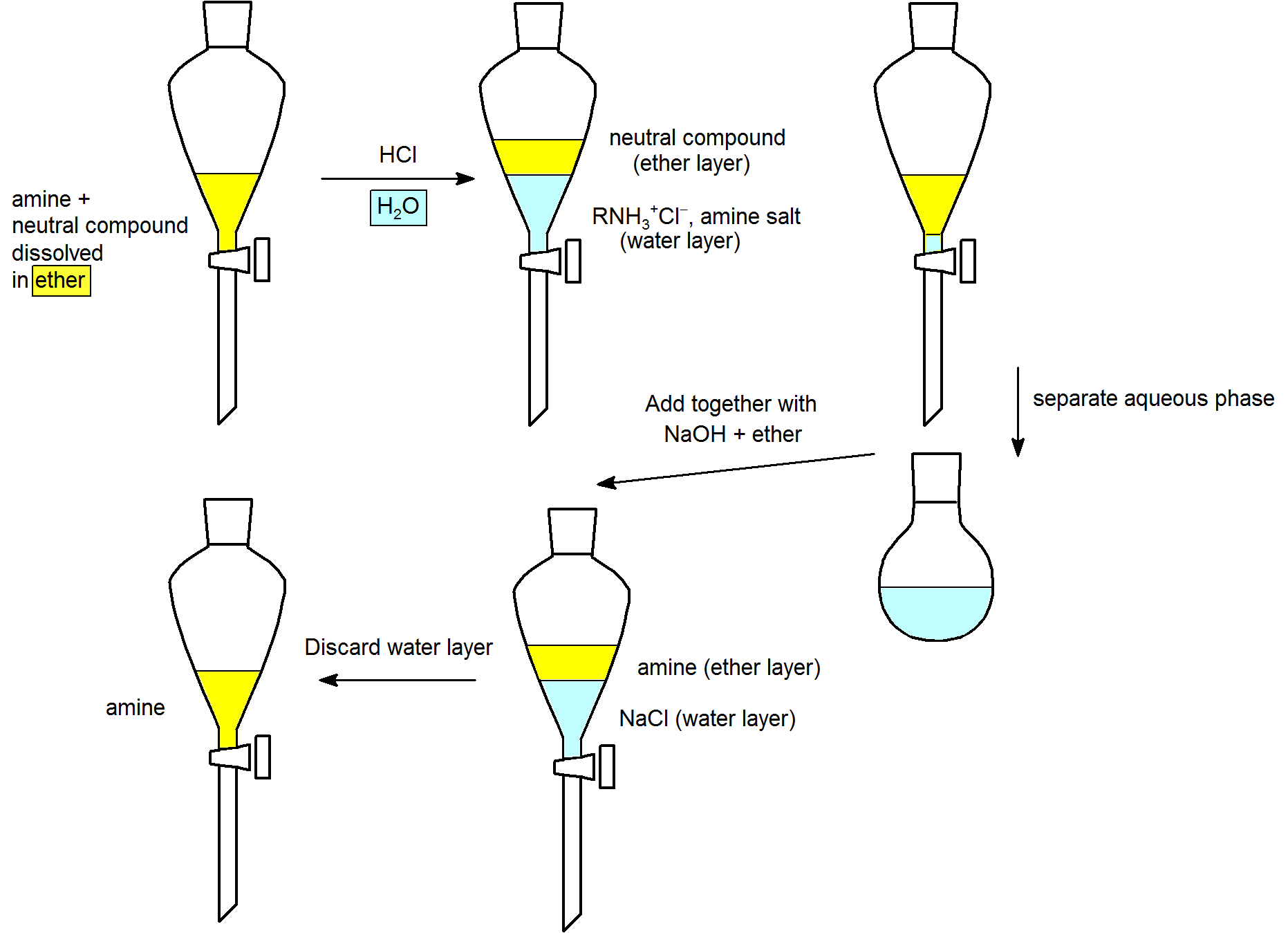## Important Reagent Bases

The significance of all these acid-base relationships to practical organic chemistry lies in the need for organic bases of varying strength, as reagents tailored to the requirements of specific reactions. The common base sodium hydroxide is not soluble in many organic solvents, and is therefore not widely used as a reagent in organic reactions. Most base reagents are alkoxide salts, amines or amide salts. Since alcohols are much stronger acids than amines, their conjugate bases are weaker than amine bases, and fill the gap in base strength between amines and amide salts.

 Base Name Formula pKa of conjugate acid Pyridine Triethyl Amine Hünig's Base Barton's Base Potassium t-Butoxide Sodium HMDS LDA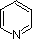(C2H5)3N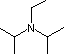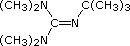(CH3)3CO(–) K(+) [(CH3)3Si]2N(–) Na(+) [(CH3)2CH]2N(–) Li(+) 5.3 10.7 11.4 14 19 26 35.7

Basicity of common amines (pKa of the conjugate ammonium ions)

Pyridine is commonly used as an acid scavenger in reactions that produce mineral acid co-products. Its basicity and nucleophilicity may be modified by steric hindrance, as in the case of 2,6-dimethylpyridine (pKa=6.7), or resonance stabilization, as in the case of 4-dimethylaminopyridine (pKa=9.7). Hünig's base is relatively non-nucleophilic (due to steric hindrance), and is often used as the base in E2 elimination reactions conducted in non-polar solvents. Barton's base is a strong, poorly-nucleophilic, neutral base that serves in cases where electrophilic substitution of other amine bases is a problem. The alkoxides are stronger bases that are often used in the corresponding alcohol as solvent, or for greater reactivity in DMSO. Finally, the two amide bases see widespread use in generating enolate bases from carbonyl compounds and other weak carbon acids.

In addition to acting as a base, 1o and 2o amines can act as very weak acids. Their N-H proton can be removed if they are reacted with a strong enough base. An example is the formation of lithium diisopropylamide (LDA, LiN[CH(CH3)2]2) by reacting n-butyllithium with diisopropylamine (pKa 36) (Section 22-5). LDA is a very strong base and is commonly used to create enolate ions by deprotonating an alpha-hydrogen from carbonyl compounds (Section 22-7).